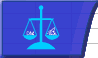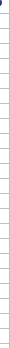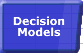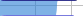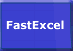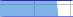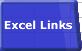# Excel Calculation MethodsSmart Recalculation Evaluation Circumstances Calculation Process Dependency Trees Controlling Calculation "CALCULATE" in Status Bar Calculation Methods Calculating from VBA Volatile Functions & Actions User-Defined Functions Repetitive Calculation Version Timing Comparison## Calculation Methods

For information on the calculation methods you can use from VBA see Calculating from VBA

### Full Calculation and Re-Calculation.

Normally Excel calculates each cell as you enter it, and minimises calculation by only recalculating all the dependents of all the changed cells in all the open Workbooks.
You can request a full calculation (all formulae) by pressing Ctrl-Alt-F9, or a recalculation (all new/changed/volatile formulae, cells and their dependents) by pressing F9.
Usually (but not always) a recalculation is faster than a full calculation.
In Excel 2002 you can request a full calculation with dependency tree rebuild by pressing Ctrl-Alt-Shift-F9.

### Sheet Calculation, Range Calculation, Formula and Part Formula Calculation.

Excel has finer levels of calculation than just the workbook:

#### Recalculate Selected Worksheet(s) (Shift-F9)

Calculate Worksheet usually flags the Workbook as uncalculated in Manual mode.

#### In Manual Mode:

Recalculates only the uncalculated and volatile cells on the selected worksheet in dependency chain sequence, or if multiple worksheets are selected they are all calculated.
Note that this only gives you "correct" results if all precedent inter-sheet and inter-workbook dependencies have already been fully calculated, and that cells on other worksheets that are dependent on the sheet(s) are not recalculated.
After a sheet calculate the formulae in the sheet, the sheet and the workbook are flagged as uncalculated (CALCULATE shows in the statusbar), unless there were no uncalculated or volatile cells. Sheet Calculate does NOT reset dirtied/uncalculated cells as calculated after calculating them: the second of two successive Shift-F9 sheet recalculates in Manual mode will calculate exactly the same cells as the first.

#### In Automatic Mode:

Recalculates all uncalculated and volatile cells on the selected worksheet, or if multiple worksheets are selected they are all calculated. If any cells were recalculated then flags the workbook as uncalculated, which triggers an automatic recalculation to recalculate any dependents on other sheets and all volatile cells and their dependents on ALL worksheets, not just the selected sheet(s). If there are no uncalculated dependents on other sheets or volatile cells then nothing happens in the triggered automatic calculation because the workbook was already calculated.

#### Calculate Selected Range (using Range Calculate in VBA)

Calculate Range resets the calculation sequence for the selected range, and flags the calculated cells as uncalculated.

#### In Manual Mode:

Calculates all the cells in the selected range left-to-right and top-to-bottom. Excel 97 and Excel 2000 ignore dependencies within the selected range, but Excel 2002 and Excel 2003 follow the left-to-right and top-to-bottom calculation with a dependency recalculation of the selected range only.

This means that range.calculate can be significantly slower in Excel 2002 and 2003 than in Excel 97 and 2000, and will give different results if there are dependencies within the range being calculated that are not left-to-right and top-to-bottom.

Excel 2007 has two Range Calculate methods: Range.Calculate, which works the same way as Excel 2002/2003, and Range.CalculateRowMajorOrder, which works the same way as Excel97/2000.

Dependents and volatile cells which are outside the range are ignored. If one of the selected cells is part of a multi-cell array formula, then all the cells in the multi-cell array formula are calculated.

• If more than one area is selected, each area is calculated left-to-right and top-to-bottom, in the sequence that the areas were selected.
• If more than one Worksheet is selected then only the selected range on the active Worksheet is calculated.
• Conditional formats are NOT evaluated.
• After the cells have been calculated they are flagged as uncalculated.
• Iteration is ignored: the calculation is only done once regardless of the Iteration settings, unless calculation mode is Automatic in which case the calculation is done once follwed by an automatic calculation according to the Iteration settings.

Range.Calculate always calculates DATEVALUE using MMDDYY format, and does not calculate conditional formats.

Although Excel 2000 SR1 is documented as containing a fix for Range Calculate it still works the same way as above.

#### In Automatic Mode:

Range Calculate triggers an an automatic recalculation but does not itself explicitly calculate the cells referred to by the range.calculate. If there are no volatile cells then no calculations are done because the workbook was already calculated.
If there are any volatile cells then ALL the volatile cells and their dependents on ALL worksheets are recalculated.

#### Excel 2002/2003 Range Calculate Problems:

Range Calculate will fail in Excel 2002/2003 if any of these conditions is true:

• Calculation is set to Manual and Iteration is enabled.
• The range being calculated contains a complete circular reference chain of formulae (you get an error message with Excel 2002 SP3 and later)
• The range being calculated partially intersects a multi-cell array formula.
• The Range.Calculate or the Selection.Calculate Methods Fail After You Update Links to a Dynamic Data Exchange (DDE) Server.
see MSKB 823338
• re-entrant calculation is not allowed: you cannot call Range.Calculate whilst a calculation is in progress
• range.calculate will fail if multiple sheets are selected

If the range you calculate includes a multi-cell array formula and you subsequently recalculate, the cells in the multi-cell array formula will be individually calculated.

#### Calculating a Single Formula or Part Formula or Array Formula

Select the formula in the Formula Bar or highlight a part of the formula in the Formula Bar and press F9.
The formula or part formula is replaced by the result.
For an array formula you will see an array of results, which is a wonderful way of debugging array formulae!
If you press Ctrl-Z (Undo) or Esc then the formula re-appears, but if you press Enter the formula or part formula is permanently replaced.

Re-entering the = sign on the front of a block of formulae will make Excel recalculate them in a similar way to Range.calculate, except that the dependency tree for the formulae will also be rebuilt and in Excel2002 and 2003 the dependency recalculation of the range will not be done in Manual mode.

#### Step-by Step Formula Evaluation in Excel 2002/2003

Excel 2002 & 2003 has an Evaluate Formula button that allows you to watch as Excel steps through calculating a formula in the selected cell.© 2001-2020 Decision Models Legal Disclaimer Privacy Statement